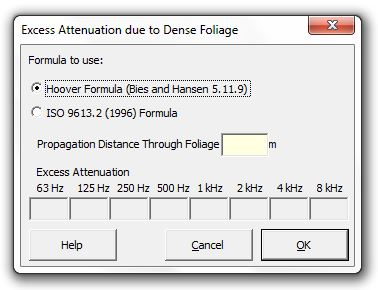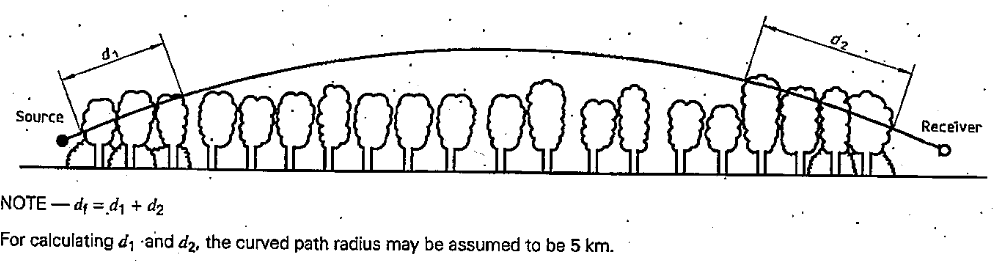### Strutt Help

Excess Attenuation due to Dense Foliage    1/1

Strutt|Environmental Noise|Dense Foliage Excess Attenuation inserts attenuation (dB) into the active row of the worksheet.Attenuation due to forests and dense foliage can be calculated using ISO 9613-2 or the Hoover formula (Bies and Hansen).

The Hoover formula calculates the attenuation using:

A_f = 0.01 * r_f(m) * f_(1/3)

Where,

f is the frequency of the propagating sound, Hz
r_f(m) is the distance of travel through the forest.

The ISO 9613-2
formula calculates the attenuation using the values in Table A.1 (ISO 9613-2). The propagation distance, df, is calculated as:References:

• Bies, D., Hansen, C., Engineering Noise Control, p227
• ISO 9613-2 Acoustics - Attenuation of sound during propagation outdoors - Part 2: General method of calculation, p.15